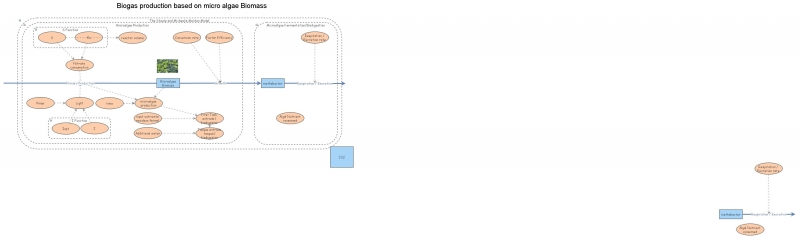Clone of Clone3f micro algae , biogas and bioelectricitySimple model to illustrate a simple simulation of the microalgae biomass production, focusing on the dependent variables such as light, nutrients and other factor that is running for a yearly period.

The biomass model uses an example, Phytoplankton growth based on Steele's and Michaelis-Menten equations), where:

Primary Production=(([Pmax]*[I]/[Iopt]*exp(1-[I]/[Iopt])*[S])/([Ks]+[S]))

Pmax: Maximum production (d-1)
I: Light energy at depth of interest (uE m-2 s-1)
Iopt: Light energy at which Pmax occurs (uE m-2 s-1)
S: Nutrient concentration (umol N L-1)
Ks: Half saturation constant for nutrient (umol N L-1).

Once this is understood, it looks upon the viability of biogas production from the microalgae biomass.